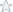# MAT 251 General Calculus - Final Exam, Midterm, Unit 5 Exam, Unit 6 Exam, Unit 7 Exam

Basically all the questions to the Final Exam, Midterm, Unit 5 Exam, Unit 6 Exam, Unit 7 Exam! (See pdf files)

Please note I did not get full marks on every test/exam but the overall average was 80%+. So do read the results carefully before purchase!

Sample Questions:

Unit 5 Exam
What Is the difference between an Indefinite Integral and a definite Integral?

Decide which trigonometric substitution on the right could be used to evaluate each of the
integrals on the left.

Match each of the Integrals on the left with the corresponding solution on the rlght

A function can have more than one anti derivative.

Unit 6 Exam
If an object is displaced a distanced by a constant force F, then the amount of work done
is given by the equation:

Suppose that f(x) is the probability density function describing the distribution for the
number of minutes H takes to solve a puzzle. Which of the following represents the probability that you with solve the puzzle in 8-10 minutes?

Which of the following differential equations is NOT separable?

Lots more. See pdfs

PDF file contains answers to the computer based test!

Topic: MAT 251 Final Exam, Midterm, Unit 5 Exam, Unit 6 Exam, Unit 7 Exam
Level: College / University
Tags:

### straighterline, blackboard, MAT251 General Calculus

Price\$9.95

Math Genius
 Shirley B. Member Since: Nov 1998 Customer Rating: 97.8% Projects Completed: 2378 Total Earnings: *Private* +1 Ratings from clients: 578
Project Details
Topic: MAT 251 Final Exam, Midterm, Unit 5 Exam, Unit 6 Exam, Unit 7 Exam
Level: College / University
Tags:

## straighterline, blackboard, MAT251 General Calculus

Customer Reviews
Rated 66 Times
Rating( 4.08 / 5 Stars)

Reviews of the solutions left by other users
No customer has left a detailed review for these math solutions. Be the first one to purchase them and leave a review for others!

Not exactly what you are looking for?
We regularly update our math homework solutions library and are continually in the process of adding more samples and complete homework solution sets. If you do not find what you are looking for, just go ahead and place an order for a custom created homework solution. You can hire/pay a math genius to do your homework for you exactly to your specifications.